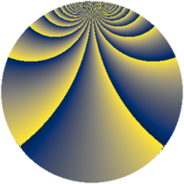# Properties

 Label 1152.3.xLevel $1152$ Weight $3$ Character orbit 1152.x Rep. character $\chi_{1152}(17,\cdot)$ Character field $\Q(\zeta_{8})$ Dimension $128$ Sturm bound $576$

# Learn more

## Defining parameters

 Level: $$N$$ $$=$$ $$1152 = 2^{7} \cdot 3^{2}$$ Weight: $$k$$ $$=$$ $$3$$ Character orbit: $$[\chi]$$ $$=$$ 1152.x (of order $$8$$ and degree $$4$$) Character conductor: $$\operatorname{cond}(\chi)$$ $$=$$ $$96$$ Character field: $$\Q(\zeta_{8})$$ Sturm bound: $$576$$

## Dimensions

The following table gives the dimensions of various subspaces of $$M_{3}(1152, [\chi])$$.

Total New Old
Modular forms 1600 128 1472
Cusp forms 1472 128 1344
Eisenstein series 128 0 128

## Trace form

 $$128q + O(q^{10})$$ $$128q - 512q^{55} - 128q^{61} + 128q^{67} - 384q^{91} + O(q^{100})$$

## Decomposition of $$S_{3}^{\mathrm{new}}(1152, [\chi])$$ into newform subspaces

The newforms in this space have not yet been added to the LMFDB.

## Decomposition of $$S_{3}^{\mathrm{old}}(1152, [\chi])$$ into lower level spaces

$$S_{3}^{\mathrm{old}}(1152, [\chi]) \cong$$ $$S_{3}^{\mathrm{new}}(96, [\chi])$$$$^{\oplus 6}$$$$\oplus$$$$S_{3}^{\mathrm{new}}(288, [\chi])$$$$^{\oplus 3}$$$$\oplus$$$$S_{3}^{\mathrm{new}}(384, [\chi])$$$$^{\oplus 2}$$